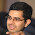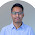## Saturday, 11 August 2012

### Pieces of a Stone

Consider a stone of 'n' kg. What is the minimum number of pieces should the stone be broken so that you can measure all the weights from 1 to 'n' kg in a weighing balance (taraju)? What should be the weight of the each individual piece?

#### 3 comments:

1.Let m be the minimum number of pieces required. Then m is the least positive integer such that 3^m >= 2n+1.

Let w_1,w_2,...,w_m be the weights required. Then w_i = 3^(i-1) for i = 1,2,...,(m-1) and w_m = n-(sum of all other weights).

PROOF:- Let us say n be the largest weight I can form using m weights (sum = n and each w_i is integer ). Then 3n+1 is the largest weight that can be formed using m+1 weights. This is because using the previous m weights, n positive and n negative weights can be formed which when combined with a weight of 2n+1 gives all weights in the range n+1 to 3n+1. Denote by M_n, the minimum number of weights required to measure all the weights between 1 and n. from the above discussion, we can say that M_(3n+1) = M_n + 1. Also, M_1 = 1. So, M_n = 2 for n = 2,3,4.....` The rest is trivial.

2.log2(n)
break the stone into weights w_i's with weight being..
w_i= ((2^i)-1)

3.The answer by Manoj is correct.
@Anshul: Try your solution with a base case of 13kg weight. It won't work. Try using Manoj's approach